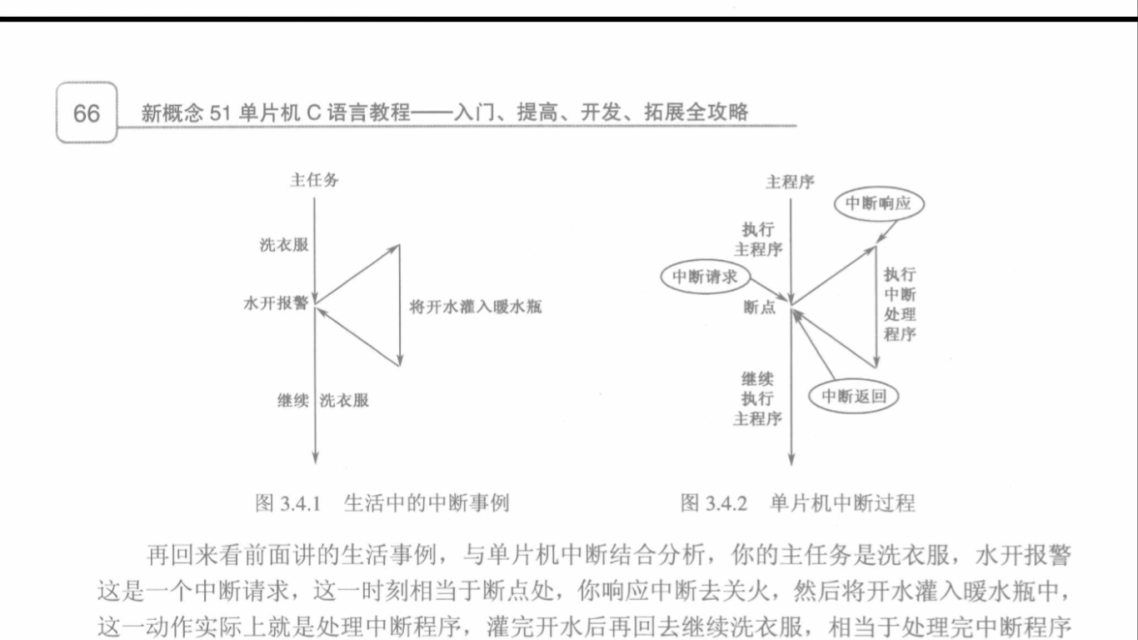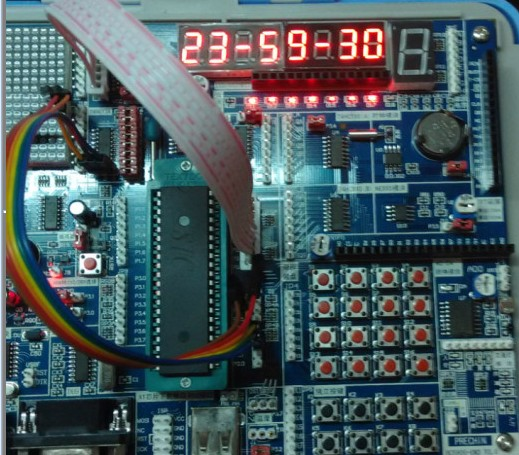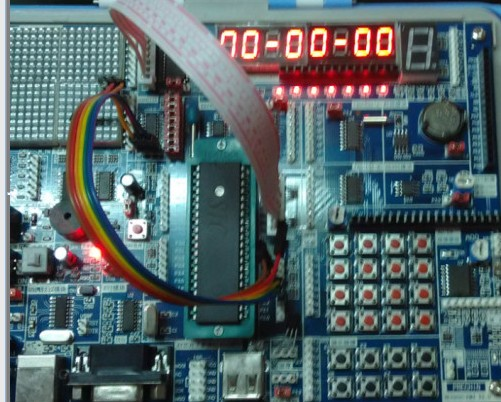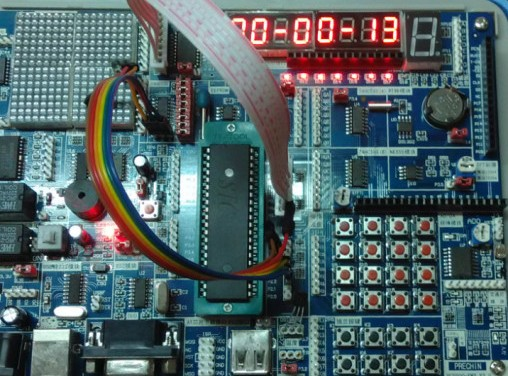# 自学单片机编程之中断与时钟程序

不喜勿喷！有好的建议或资料请@我！#include<reg51.h>    //51系列单片机头文件

#define uchar  unsigned char   //宏定义

#define ab   P0

//--定义全局变量--//

uchar        int_time;

uchar    a,b,c;

sbit  duanA=P2^2;

sbit  duanB=P2^3;

sbit  duanC=P2^4;

unsigned  char  code  table[]={0x3f,0x06,0x5b,0x4f,0x66,0x6d,0x7d,0x07,

0x7f,0x6f,0x77,0x7c,0x39,0x5e,0x79,0x71};  //0123456789AbCdEF的显示码

uchar DisplayData;             //用来存放要显示的8位数的值

void Digdisplay();                 //--声明全局变量--//

/****************************中断子程序*******************************/

void  clock(uchar  a,b,c)

{

DisplayData=table[c/10];

DisplayData=table[c%10];

DisplayData=0x40;

DisplayData=table[b/10];

DisplayData=table[b%10];

DisplayData=0x40;

DisplayData=table[a/10];

DisplayData=table[a%10];

Digdisplay();

}        对于上面的绿底的时钟程序包括一些有点基础看的不是很懂，因为我的开发板上有138译码器。上面的程序用译码器的原理控制的8个数码管而不是P2这个I/O端口，它完全可以替换成用P2端口控制的。可参照我的周记二来写。上面就用到了简单运算来改变参数如C/10C=10则等于1/是运算符号除法取商，%是除法取余；

/*****************************中断主程序*****************************/

void  main()

{

TMOD=0x01;                                 //设定使用定时器0方式

TH0=(65536-46083)/256;                                                        //装初值

TL0=(65536-46083)%256;                                                       //...

EA=1;                                                                                            //打开全局中断控制

ET0=1;                                                                                          //打开定时器0中断

TR0=1;                                                                                         //启动定时器0

int_time=0;                                                                                 //中断计数变量初始值

a=0;                                                                            //秒计数变量初始值

b=59;                                                                                   //分计数变量初始值

c=23;                                                                         //时计数变量初始值

while(1)                                //程序在这里不停的对数码管动态扫描同时等待中断发生

{

clock(a,b,c);        //调用中断日期子程序

}

}上面程序中红色的程序用到的定时器只是其中一种，定时器的使用方式也是。具体的中断比这复杂许多，详情请参照相关书籍！

void Digdisplay()

{

uchar i,j;

for(i=0;i<8;i++)

{

switch(i)                      //位选，选择点亮的数码管，

{

case 0:

duanA=0;duanB=0;duanC=0;break; //显示第0

case 1:

duanA=1;duanB=0;duanC=0;break; //显示第1

case(2):

duanA=0;duanB=1;duanC=0;break; //显示第2

case(3):

duanA=1;duanB=1;duanC=0;break; //显示第3

case(4):

duanA=0;duanB=0;duanC=1;break; //显示第4

case(5):

duanA=1;duanB=0;duanC=1;break; //显示第5

case(6):

duanA=0;duanB=1;duanC=1;break; //显示第6

case(7):

duanA=1;duanB=1;duanC=1;break; //显示第7

}

ab=DisplayData[ i];            //发送段码

j=50;                                                        //扫描间隔时间设定

while(j--);

ab=0x00;            //消隐

}

}

/*************************定时器T0的中断服务子程序*********************/

void T0_time() interrupt 1

{    // uchar a,b,c;

TH0=(65536-50000)/256;         //重装初值

TL0=(65536-50000)%256;         //重装初值

int_time++;

if(int_time==20)                                   //如果到了20次，说明1s时间到

{

int_time=0;

a++;

}

if(a==60)

{

b++;

a=0;

}

if(b==60)

{

c++;

b=0;

}

if(c==24)

{

c=0;

}

}if(a==60)

{

b++;

a=0;

}

if(b==60)

{

c++;

b=0;

}

if(c==24)

{

c=0;

}

##### Tags：中断,时钟程序,单片机

• 上一篇文章：
• 下一篇文章： 没有了
• 请文明参与讨论，禁止漫骂攻击，不要恶意评论、违禁词语。 昵称：
 1分 2分 3分 4分 5分 还可以输入 200 个字
网友评论
推荐文章
最新推荐
热门文章